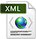Partnerships and Cooperations
BibliographyPDF e-Pub

## Section: New Results

### On the index of multi-mode DAE Systems

Hybrid systems modelers exhibit a number of difficulties related to the mix of continuous and discrete dynamics and sensitivity to the discretization scheme. Modular modeling, where subsystems models can be simply assembled with no rework, calls for using Differential Algebraic Equations (DAE). In turn, DAE are strictly more difficult than ODE. In most modeling and simulation tools, before simulation can occur, sophisticated pre-processing is applied to DAE systems based on the notion of differentiation index. Graph based algorithms such as the one originally proposed by Pantelides   are efficient at finding the differentiation index of a DAE system, structurally (i.e., outside some exceptional values for the system parameters), solving the consistent initialisation problem and, transforming a DAE system into a statically scheduled system of ordinary differential equations (ODE) and implicit functions. The differentiation index for DAE explicitly relies on everything being differentiable. Therefore, extensions to hybrid systems must be done with caution — to our knowledge, no such extension exists, supported by a rigourous mathematical theory. In  , we use non-standard analysis for this. Non-standard analysis formalizes differential equations as discrete step transition systems with an infinitesimal time basis. This allows to map hybrid DAE systems to difference Algebraic Equations (dAE), for which the notion of difference index can be used. The difference index of a dAE is an easy transposition of the differentiation index of a DAE, where forward shift in time (using a $\text{next}\left(\right)$ operator) replaces differentiation. We prove that the differentiation index of a DAE is structurally equal to the difference index of the dAE resulting from its non-standard interpretation. We can thus propose the difference index of the non-standard semantics of a hybrid DAE system, as a consistent extension of both the differentiation index of DAE and the difference index of dAE. It turns out that the index theory for (discrete time) dAE systems is interesting in itself and raises new issues. We have investigated graph based method similar to the Pantelides   algorithm for computing the difference index of a dAE.